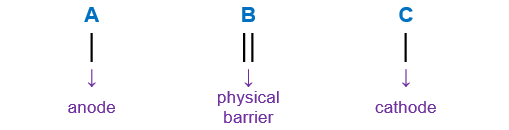# Problem: The voltage generated by the zinc concentration cell described by,Zn(s) | Zn2+ (aq, 0.100 M) || Zn2+ (aq, ? M) | Zn(s)is 18.0 mV at 25°C.

###### FREE Expert Solution

Zn(s) | Zn2+ (aq, 0.100 M) || Zn2+ (aq, ? M) | Zn(s)

When writing a cell notation, we use the following format – “as easy as ABCLose               Gain
Electron         Electrons
Oxidation       Reduction

lose electrons oxidation → anode
gain electrons reduction → cathode

90% (205 ratings)###### Problem Details

The voltage generated by the zinc concentration cell described by,

Zn(s) | Zn2+ (aq, 0.100 M) || Zn2+ (aq, ? M) | Zn(s)

is 18.0 mV at 25°C.